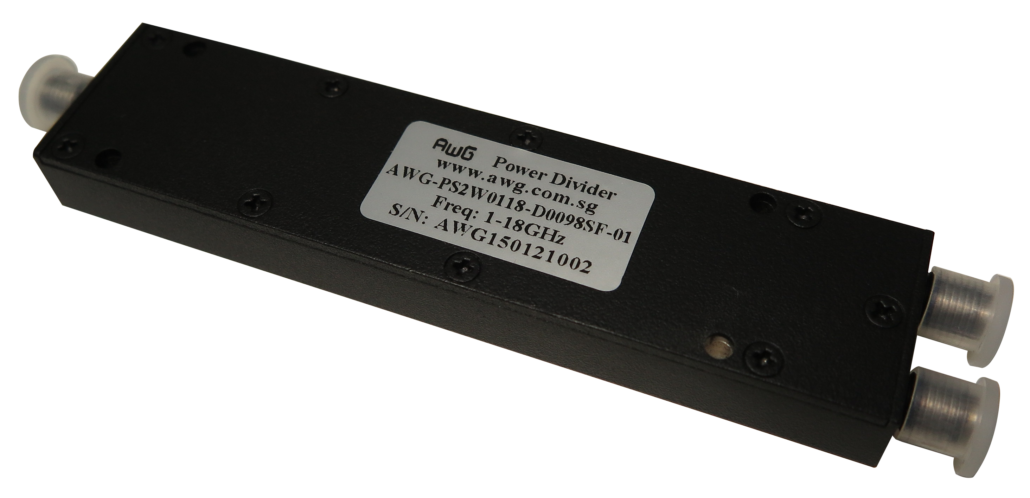# Wideband Power Divider## Wideband Power Divider: What is it?

A typical single stage Wilkinson power divider is considered a narrowband power divider. This is because the isolation and the return loss of the power divider degrades as the frequency deviates from the center frequency of the device. We normally use the 10dB guideline to determine the bandwidth of the power divider. Thus the operating bandwidth is the bandwidth in which the return loss and isolation drops to 10dB.

Whilst narrowband power divider has its uses, many systems nowadays require broader bandwidth and hence a narrowband power divider may no longer be useful. One typical example of a wideband power divider is APS-2S-700M-2700M-01 which can operate from 700MHz to 2700MHz, giving an operational bandwidth of 2GHz. With a center frequency of 1.7GHz, this power divider has more than 100% fractional bandwidth. This power divider is typically used in mobile communications whereby there is a need to serve the low end of 700MHz (for LTE systems) up to 2.7GHz (for EDGE).

### What are the types of wideband power divider that AWG Tech has?

AWG Tech specializes in wideband and ultra wideband power dividers. Some of the common wideband power dividers are:

• 1 to 18 GHz power divider (2 way. 3 way. 4 way. 8 ways)
• 2 to 18 GHz power divider
• 700 to 2700 MHz power divider
• 6 to 18 GHz power divider
• 1 to 4GHz power divider.

The above power dividers are also available in 2-way, 3-way, 4-way, 8-way configuration

## The Basics of a Wideband Power Divider

Power dividers are passive RF components that divide an input signal frequency signal into two or more output frequencies with minimal losses. They are widely utilized in wireless systems to split the power equally across the system. The signals that are produced by these components are typically of the same amplitude and phase.

However, based on the requirements, dividers can vary the amplitude and phase of the signal outputs. In addition, dividers can also be utilized as either a power combiner or a power divider; in other terms, it’s a reciprocal component. There are different types, each having their own functions. One major example would be the wideband power divider.

## What is a Wideband?

To better understand the function of the above mentioned RF component, it’s better to understand what the wideband is all about first.

In communications, a system is considered wideband when the message bandwidth significantly surpasses the coherence bandwidth of the channel. There are some communication links that have a very high data rate that they are forced to utilize a wide bandwidth.

However, there are other links that may have relatively low rates of data; but deliberately utilize a much wide bandwidth than “needed” for that data rate in order to gain other benefits.

In addition, a wideband antenna is one with approximately or precisely the same functioning traits over a very wide passband. It’s determined from the broadband antennas where the passband is large. However, the antenna gain and/or radiation pattern doesn’t need to stay the same over the passband.

The phrase “wideband audio”, also referred to as HD Voice or Wideband Voice, denotes a telephony utilizing a wideband codec. This utilizes a greater frequency ranges of the audio spectrum than the traditional voice band telephone call, thus resulting in a clearer audio.

In this context, the wideband is usually referred to as cover frequencies in the range of 50 – 7,000Hz, thus permitting audio with richer tones and of high-quality.

### The Power Divider Key Specifications

Just like with any other RF devices, the power divider also comes with its own major specifications. Listed below are just some of the key specifications one must need to know about:

• The Configuration: This refers to the number of methods the input signal is divided by the power divider.
• The Power: This pertains to the average input power that the power divider can hold.
• The Insertion Loss: This refers to the signal that’s lost when a single signal is being transformed into multiple signals is referred to as insertion loss, it’s usually measured in dB.
• The Package Type: Most of the time, power dividers are usually available in a number of packages. There are some types that include a module with connectors, surface mount, a plug-in, a flat pack and drop ins.
• The Impedance: Power dividers are generally available in either 50 Ohms and 75 Ohms configurations.

These are just some of the important fundamentals one must learn about when using a wideband directional coupler. Should more questions arise and answers can’t be found here, our RF engineers are always ready to answer all inquiries.

4 + 3 = ?